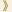HomeShaw Laureates2018Mathematical SciencesPress Release

The Shaw Prize in Mathematical Sciences 2018 is awarded to Luis A Caffarelli, Professor of Mathematics at the University of Texas at Austin, USA for his groundbreaking work on partial differential equations, including creating a theory of regularity for nonlinear equations such as the Monge–Ampère equation, and free-boundary problems such as the obstacle problem, work that has influenced a whole generation of researchers in the field.

Partial differential equations are fundamental to large parts of mathematics, physics, and indeed all the sciences. They are used to model heat flow, fluid motion, electromagnetic waves, quantum mechanics, the shape of soap bubbles, and innumerable other physical phenomena.

A few very simple equations can be solved explicitly — that is, one can find an exact formula for their solutions — but this is very much the exception rather than the rule. Instead, one has to be content with being able to show that solutions exist, and with being able to say something about how they behave.

A very important example of this is the Navier–Stokes equation, which describes the motion of a viscous fluid. It is not known whether, given appropriate initial conditions, there must be a solution to the Navier–Stokes equation that remains well-behaved forever, or whether singularities will necessarily develop. To put it more graphically, if you stir a bucket of water, is there a danger that a week later it will blow up? Probably not, but nobody knows how to prove this, and it is one of the major unsolved problems of mathematics.

Although it is not known how to solve the Navier–Stokes equations, one can find so-called “weak solutions”, which are abstract objects that solve the equations, but not in quite the sense one wants. If one could show that these solutions were “regular”, then the Navier–Stokes problem would be solved. A famous result of Caffarelli, Kohn and Nirenberg is the closest anybody has come to that: it shows that weak solutions exist that are regular except on a set of singularities that has to be very small, in a precise mathematical sense.

Another area in which Caffarelli has created a new and highly influential theory is obstacle problems. Here one would like to know the shape that will be taken by an elastic membrane with a given boundary if it has to lie above a certain obstacle. The shape taken will be the one that minimizes its energy, but the important questions concern how well-behaved, or “regular”, a solution of this kind will be. As with all important problems in partial differential equations, this one arises in many contexts, including fluid filtration in porous media, and financial mathematics.

In general, because one does not usually have explicit formulae for solutions to partial differential equations, the analysis of their properties is very hard, and depends on extremely delicate estimates. Caffarelli is a master at this, frequently coming up with arguments that have left other researchers wondering how he could possibly have thought of them. He continues to work at the forefront of the field and has had a huge influence, both through his own work and that of his doctoral students, many of whom have themselves become extremely distinguished mathematicians. In a way that few mathematicians achieve even once, he has repeatedly created important areas almost from scratch that are extremely active to this day.

Mathematical Sciences Selection Committee
The Shaw Prize

14 May 2018  Hong Kong